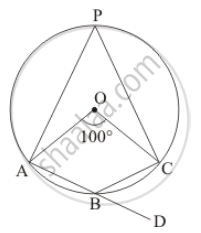Advertisement Remove all ads

# In the Given Figure, O is the Centre of the Circle. Find ∠Cbd. - Mathematics

Short Note

In the given figure, is the centre of the circle. Find ∠CBD.Advertisement Remove all ads

#### Solution

It is given that, angle AOC = 100°We have to find  angleCBD

Since   angleAOC = 100°     (Given)

So,

angleAPC = 1/2 angleAOC        (The angle subtended by an arc of a circle at the centre is double the angle subtended by it at any point on the remaining part of the circle.)

⇒ angleAPC = 1/2 xx 100

= 50°

Now,
angle APC + angleABC = 180°
(Opposite pair of angle of cyclic quadrilateral)

So,

50°+ angleABC = 180°

angleABC = 180° - 50°

= 130°

⇒angle ABC  =  130°      …… (1)

angle ABC + angleCBD = 180°     (Linear pair)

130° + angle CBD = 180°       ( angleABC = 130)

angle CBD = 180° - 130°

= 50°

Hence  angle CBD  = 50°

Is there an error in this question or solution?
Advertisement Remove all ads

#### APPEARS IN

RD Sharma Mathematics for Class 9
Chapter 15 Circles
Exercise 15.5 | Q 6 | Page 101
Advertisement Remove all ads
Advertisement Remove all ads
Share
Notifications

View all notifications

Forgot password?
Course